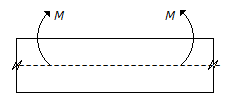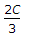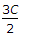# Mechanical Engineering - Strength of Materials

### Exercise :: Strength of Materials - Section 7

36.

For a given stress, the ratio of moment of resistance of a beam of square cross-section when placed with its two sides horizontal to the moment of resistance with its diagonal horizontal, is

 A. 1/2 B. 1 C. 1/2 D. 2

Explanation:

No answer description available for this question. Let us discuss.

37.

The Young's modulus of a material is 125 GPa and Poissons ratio is 0.25. The modulus of rigidity of me material is

 A. 30 GPa B. 50 GPa C. 80 GPa D. 100 GPa

Explanation:

No answer description available for this question. Let us discuss.

38.

The layer at the centre of gravity of the beam as shown in the below figure, will beA. in tension B. in compression C. neither in tension nor in compression

Explanation:

No answer description available for this question. Let us discuss.

39.

If the modulus of elasticity for a given material is twice its modulus of rigidity, then bulk modulus is equal to

 A. 2C B. 3C C.D.Explanation:

No answer description available for this question. Let us discuss.

40.

A simply supported beam 'A' of length l, breadth b and depth d carries a central point load W. Another bream 'B' has the same length and breadth but its depth is doubled. The deflection of beam 'B' will be double as compared to beam 'A'.

 A. Correct B. Incorrect